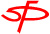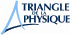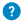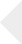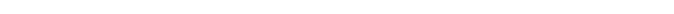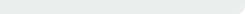The Meissner effect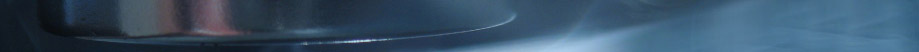# The Meissner effect

## Magnetic field expeled

Some materials tend to expel the magnetic field; these materials are said to be “diamagnetic”, but the effects are very weak. For instance, water and the human body are diamagnetic materials. However, because of the Meissner effect, the superconducting material creates currents which completely oppose the magnetic field applied by a magnet. A superconductor in a Meissner state is hence a perfect diamagnet.

The currents responsible for this effect do not need any energy to continue, but the system must apply the initial energy to make them accelerate until they reach the value that enables the cancellation of the external magnetic field. If the magnetic field to be expelled is too strong, the system will not be able to develop the necessary supercurrents; therefore there will be no superconductivity and the sample will simply behave as a normal metal. The critical magnetic field over which the material will not become superconducting even when cooled is called Bc. Superconductivity depends on both the temperature and the magnetic field, which can be represented on a phase diagram.

Be careful, the Meissner effect is different from induction! Indeed, the classic laws of electromagnetism enable a magnetic field that is not equal to zero to go through a perfect metal (R = 0).  In such a case, the variation of the magnetic field is not permitted (dB/dt = 0). However, in the Meissner effect, the magnetic field does not change in time; the magnet remains the same, and yet it starts to levitate.

In order to describe the properties of a superconductor and explain the Meissner effect, stating that the electric resistance is equal to zero (R = 0) as in a perfect metal is not enough. A new behavior, described by the London equation, which replaces the usual Ohm’s law, must be introduced because the superconducting electrons do not obey the same law as the metal electrons.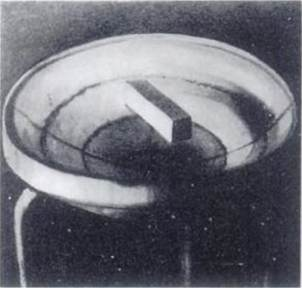Magnet levitating above a lead bowl. This is a true Meissner levitation.
Lead is a type I superconductor below a temperature of 7.2 K; in this picture, it was cooled at 4.2 K with liquid helium. Vortices cannot exist in lead, and only the concave shape of the superconductor gives the levitation stability and prevents the magnet from sliding on the side.

The superconducting state is characterized by two properties: no electric resistance (R = 0) and no magnetic field (B = 0). Those are two independent properties: neither is the consequence of the other.